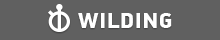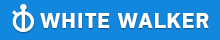GMAT Question of the Day - Daily to your Mailbox; hard ones only

 It is currently 26 Jun 2019, 11:22GMAT Club Daily Prep

Thank you for using the timer - this advanced tool can estimate your performance and suggest more practice questions. We have subscribed you to Daily Prep Questions via email.

Customized
for You

we will pick new questions that match your level based on your Timer History

Track

every week, we’ll send you an estimated GMAT score based on your performance

Practice
Pays

we will pick new questions that match your level based on your Timer History

Not interested in getting valuable practice questions and articles delivered to your email? No problem, unsubscribe here.One inlet pipe can fill an empty cistern to 1/3 of its capacity in 3

Author Message
TAGS:

Hide Tags

Math ExpertV
Joined: 02 Sep 2009
Posts: 55803
One inlet pipe can fill an empty cistern to 1/3 of its capacity in 3  [#permalink]

Show Tags00:00

Difficulty:15% (low)

Question Stats:88% (01:56) correct12% (03:06) wrongbased on 85 sessions

HideShow timer StatisticsOne inlet pipe can fill an empty cistern to 1/3 of its capacity in 3 hours. A second inlet pipe can fill the empty cistern to 3/4 of its capacity in 4.5 hours. If both pipes are opened simultaneously, how long, in hours, will it take to fill the cistern?

(A) 4.75
(B) 4.25
(C) 3.75
(D) 3.6
(E) 3.25

_________________
Math ExpertV
Joined: 02 Sep 2009
Posts: 55803
Re: One inlet pipe can fill an empty cistern to 1/3 of its capacity in 3  [#permalink]

Show Tags

Bunuel wrote:
One inlet pipe can fill an empty cistern to 1/3 of its capacity in 3 hours. A second inlet pipe can fill the empty cistern to 3/4 of its capacity in 4.5 hours. If both pipes are opened simultaneously, how long, in hours, will it take to fill the cistern?

(A) 4.75
(B) 4.25
(C) 3.75
(D) 3.6
(E) 3.25

17. Work/Rate Problems

On other subjects:
ALL YOU NEED FOR QUANT ! ! !
_________________
Senior PS ModeratorD
Status: It always seems impossible until it's done.
Joined: 16 Sep 2016
Posts: 749
GMAT 1: 740 Q50 V40GMAT 2: 770 Q51 V42Re: One inlet pipe can fill an empty cistern to 1/3 of its capacity in 3  [#permalink]

Show Tags

Bunuel wrote:
One inlet pipe can fill an empty cistern to 1/3 of its capacity in 3 hours. A second inlet pipe can fill the empty cistern to 3/4 of its capacity in 4.5 hours. If both pipes are opened simultaneously, how long, in hours, will it take to fill the cistern?

(A) 4.75
(B) 4.25
(C) 3.75
(D) 3.6
(E) 3.25

IMO D.

First pipe fills 1/3rd cistern in 3 hours. So whole cistern in 9 hours. Therefor it's rate = 1/9 cistern per hour.
Second pipe fills 3/4th cistern in 4.5 hours.

So it fills 1 cistern in 4.5 * 4/3 = 6 hours. Rate of second pipe = 1/6 cistern per hour

When working together rates will add up.

together rate = 1/6 + 1/9
=3/18 + 2/18
=5/18 cistern in one hour

Hence 1 cistern in 18/5 hours or 3.6 hrs

Hence Option D.

Best,
_________________
Regards,

“Do. Or do not. There is no try.” - Yoda (The Empire Strikes Back)
Senior SC ModeratorV
Joined: 22 May 2016
Posts: 2950
One inlet pipe can fill an empty cistern to 1/3 of its capacity in 3  [#permalink]

Show Tags

Bunuel wrote:
One inlet pipe can fill an empty cistern to 1/3 of its capacity in 3 hours. A second inlet pipe can fill the empty cistern to 3/4 of its capacity in 4.5 hours. If both pipes are opened simultaneously, how long, in hours, will it take to fill the cistern?

(A) 4.75
(B) 4.25
(C) 3.75
(D) 3.6
(E) 3.25

Pipe 1 rate: $$\frac{(\frac{1}{3})}{3}=(\frac{1}{3}*\frac{1}{3})=\frac{1}{9}$$

Pipe 2 rate: $$\frac{\frac{3}{4}}{\frac{9}{2}}=(\frac{3}{4}*\frac{2}{9})=\frac{1}{6}$$

Together, combined rate is
$$(\frac{1}{9}+\frac{1}{6})=\frac{15}{54}=\frac{5}{18}$$

W = 1 (cistern)
Rate and time are inversely proportional, and here, multiplied, they must equal 1.

Flip the rate to get the time, in hours, that it would take to fill the cistern*:
$$\frac{18}{5}= 3.6$$ hours

*OR
$$R*T = W$$, so $$T=\frac{W}{R}$$
$$T = \frac{1}{(\frac{5}{18})}= (1* \frac{18}{5})=3.6$$ hours

_________________
SC Butler has resumed!
Get two SC questions to practice, whose links you can find by date, here.

Tell me, what is it you plan to do with your one wild and precious life? -- Mary Oliver
Board of DirectorsP
Status: QA & VA Forum Moderator
Joined: 11 Jun 2011
Posts: 4505
Location: India
GPA: 3.5
Re: One inlet pipe can fill an empty cistern to 1/3 of its capacity in 3  [#permalink]

Show Tags

Bunuel wrote:
One inlet pipe can fill an empty cistern to 1/3 of its capacity in 3 hours. A second inlet pipe can fill the empty cistern to 3/4 of its capacity in 4.5 hours. If both pipes are opened simultaneously, how long, in hours, will it take to fill the cistern?

(A) 4.75
(B) 4.25
(C) 3.75
(D) 3.6
(E) 3.25
First inlet pipe can fill the empty cistern in 9 hours and the second inlet pipe can fill the cistern in 6 hours.

Let the total capacity of the Cistern be 18 units.

So, Efficiency of the First Inlet pipe is 2 units/hour and efficiency of the Second inlet pipe is 3 units/hour

Combined efficiency of both the pipes is 5 units /hour, thus working together the 2 machines can fill the cistern in $$\frac{18}{5}$$ = $$3.6$$ hours, Answer must be (D)
_________________
Thanks and Regards

Abhishek....

PLEASE FOLLOW THE RULES FOR POSTING IN QA AND VA FORUM AND USE SEARCH FUNCTION BEFORE POSTING NEW QUESTIONS

How to use Search Function in GMAT Club | Rules for Posting in QA forum | Writing Mathematical Formulas |Rules for Posting in VA forum | Request Expert's Reply ( VA Forum Only )
Target Test Prep RepresentativeD
Status: Founder & CEO
Affiliations: Target Test Prep
Joined: 14 Oct 2015
Posts: 6680
Location: United States (CA)
Re: One inlet pipe can fill an empty cistern to 1/3 of its capacity in 3  [#permalink]

Show Tags

Bunuel wrote:
One inlet pipe can fill an empty cistern to 1/3 of its capacity in 3 hours. A second inlet pipe can fill the empty cistern to 3/4 of its capacity in 4.5 hours. If both pipes are opened simultaneously, how long, in hours, will it take to fill the cistern?

(A) 4.75
(B) 4.25
(C) 3.75
(D) 3.6
(E) 3.25

We see that the first pipe has a rate of (1/3)/3 = 1/9 and the second pipe has a rate of (3/4)/4.5 = (3/4)/(9/2) = 3/4 x 2/9 = 1/6. Let t = the time, in hours, it takes for both pipes work together to fill the cistern, we can create the equation:

t(1/9 + 1/6) = 1

t(2/18 + 3/18) = 1

t(5/18) = 1

t = 18/5 = 3.6

_________________

Scott Woodbury-Stewart

Founder and CEO

Scott@TargetTestPrep.com

See why Target Test Prep is the top rated GMAT quant course on GMAT Club. Read Our Reviews

If you find one of my posts helpful, please take a moment to click on the "Kudos" button.

CrackVerbal Quant ExpertB
Joined: 12 Apr 2019
Posts: 127
Re: One inlet pipe can fill an empty cistern to 1/3 of its capacity in 3  [#permalink]

Show Tags

This is a fairly simple question on rates. At your peak, you should be able to solve this question in less than a minute.

If the first inlet pipe can fill $$\frac{1}{3}$$rd of an empty cistern in 3 hours, we can conclude that it can fill the whole cistern in 9 hours.

Similarly, if the second inlet pipe can fill $$\frac{3}{4}$$th of the cistern in 4.5 hours, it can fill the whole cistern in 6 hours.

Let us assume the volume of the cistern to be 18 gallons, which is the LCM of 9 and 6. This represents the work to be done by the inlet pipes.

The first pipe can fill 18 gallons in 9 hours, so it fills at the rate of 2 gallons per hour. We can conclude similarly, that the second pipe fills at the rate of 3 gallons per hour.
If they are opened simultaneously, they can fill 5 gallons per hour.

At this rate, they will take

$$\frac{18}{5}$$ = 3.6 hours to fill the entire cistern.

The correct answer option is D.

Hope this helps!
_________________Re: One inlet pipe can fill an empty cistern to 1/3 of its capacity in 3   [#permalink] 04 Jun 2019, 08:28
Display posts from previous: Sort by

One inlet pipe can fill an empty cistern to 1/3 of its capacity in 3